# 集成学习

2019-01-10

• 并行集成方法，其中参与训练的基础学习器并行生成（例如 Random Forest）。并行方法的原理是利用基础学习器之间的独立性，通过平均可以显著降低错误。

## 1 Bagging

BaggingBootstrap Aggregating的简称，对训练数据擦用自助采样(Boostrap sampling)，即有放回地采样；每一次的采样数据集训练出一个基分类器，经过$M$次采样得到$M$个基分类器。对于训练得到的$M$个基分类器，分类问题可以采用投票方法，回归问题可以采用平均的方法来进行对测试样例的判断。具体的过程如下：

1. 从原始样本集中抽取训练集。每轮从原始样本集中使用Bootstraping（有放回）的方法抽取$n$个训练样本（在训练集中，有些样本可能被多次抽取到，而有些样本可能一次都没有被抽中）。共进行$K$轮抽取，得到$K$个训练集。（我们这里假设$K$个训练集之间是相互独立的，事实上不是完全独立）
2. 每次使用一个训练集得到一个模型$K$个训练集共得到$K$个模型。但是是同种模型。（注：$K$个训练集虽然有重合不完全独立，训练出来的模型因为是同种模型也是不完全独立。这里并没有具体的分类算法或回归方法，我们可以根据具体问题采用不同的分类或回归方法，如决策树、感知器等）
3. 分类问题：将上步得到的k个模型采用投票的方式得到分类结果；对回归问题，计算上述模型的均值作为最后的结果。（所有模型的重要性相同）
• Voting 投票策略：就是每个模型对测试样例进行投票，票数多的为测试的结果，如果出现投票数相同，要么随机选择一个，要么重新训练一个模型进行再分类。
• Average 均值策略：就是对所有的结果进行平均，一个改进的版本可以用加权的方式进行平均。例如$A$$B$$C$三个分类器得到的结果先进行排序，根据排序的不同赋予不同的权重

Bagging算法中，基分类器之间不存在依赖关系，基分类器可以并行生成。

Bagging的性能依赖于基分类器的稳定性，如果基分类器是不稳定的，bagging有助于减低训练数据的随机扰动导致的误差，但是如果基分类器是稳定的，即对数据变化不敏感，那么bagging方法就得不到性能的提升，甚至会减低。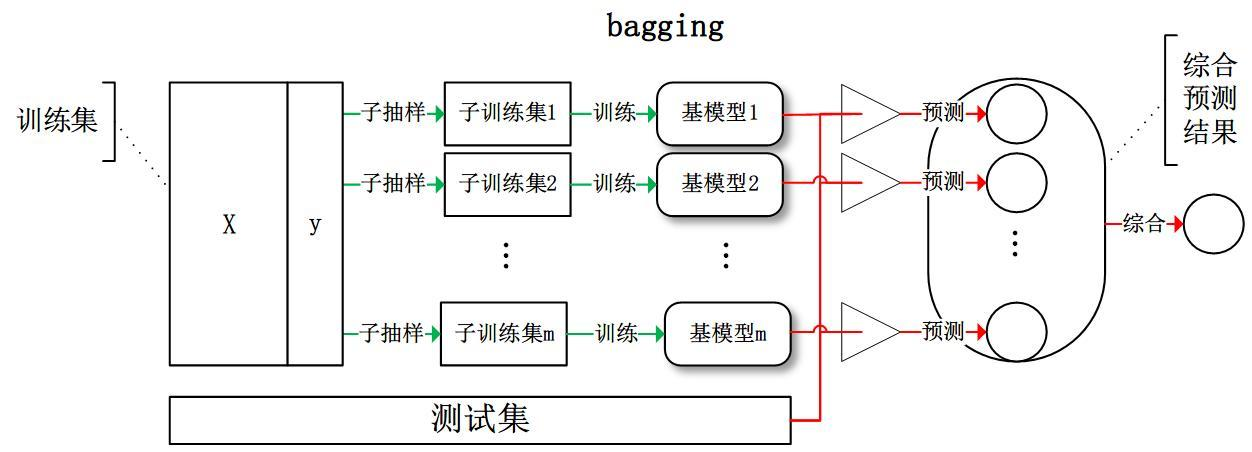### 1.1 随机森林(Random Forest)

• 采样： 首先是两个随机采样的过程，Random Forest对输入的数据要进行行、列的采样对于行采样，采用有放回的方式，也就是在采样得到的样本集合中，可能有重复的样本。假设输入样本为$N$个，那么采样的样本也为$N$个。这样使得在训练的时候，每一棵树的输入样本都不是全部的样本，使得相对不容易出现过拟合。然后进行列采样，从$M$个属性中随机选择$m$个( $m << M$ )。
• 完全分裂： 之后就是对采样之后的数据使用完全分裂的方式建立出决策树，这样决策树的某一个叶子节点要么是无法继续分裂的，要么里面的所有样本的都是指向的同一个分类。一般很多的决策树算法都一个重要的步骤——剪枝，但是这里不这样干，由于之前的两个随机采样的过程保证了随机性，所以就算不剪枝，也不会出现过拟合。

1. 假如有$N$个样本，则有放回的随机选择$N$个样本(每次随机选择一个样本，然后返回继续选择)。这选择好了的$N$个样本用来训练一个决策树，作为决策树根节点处的样本。
2. 当每个样本有$M$个属性时，在决策树的每个节点需要分裂时，随机从这$M$个属性中选取出$m$个属性，满足条件$m << M$【推荐值$m=\log_2 M$】。然后从这$m$个属性中采用某种策略（比如说信息增益）来选择1个属性作为该节点的分裂属性。
3. 决策树形成过程中每个节点都要按照步骤2来分裂（很容易理解，如果下一次该节点选出来的那一个属性是刚刚其父节点分裂时用过的属性，则该节点已经达到了叶子节点，无须继续分裂了）。一直到不能够再分裂为止。注意整个决策树形成过程中没有进行剪枝。
4. 按照步骤1~3建立大量的决策树，这样就构成了随机森林了。

• 在数据集上表现良好
• 在当前的很多数据集上，相对其他算法有着很大的优势
• 它能够处理很高维度(feature很多)的数据，并且不用做特征选择
• 在训练完后，它能够给出哪些feature比较重要
• 在创建随机森林的时候，对generlization error(泛化误差)使用的是无偏估计
• 训练速度快
• 在训练过程中，能够检测到feature间的互相影响
• 容易做成并行化方法
• 实现比较简单

• 当随机森林中的决策树个数很多时，训练时需要的空间和时间会较大
• 随机森林模型还有许多不好解释的地方，有点算个黑盒模型

## 2 Boosting

Boosting利用一种迭代的方法，每一次训练的数据都在前一次训练的基础上产生。具体来说就是在每一次训练的时候会更加关心分类错误的样例，给这些分类错误的样例增加更大的权重。然后进行迭代训练，最后将这些分类器进行加权得到最后的分类器。但是Boosting方法虽然会比Bagging更容易得到好的效果，也会存在过拟合的问题。具体过程如下：

1. 开始的时候在训练集$T$上，每一个训练样本都被赋予一个初始权重，然后训练一个弱分类器，计算该分类器的误差，然后利用该误差计算该分类器的权重系数
2. 根据上一步的结果对训练集$T$进行权值调整，训练集$T$中数据被赋予新的权值：对错分的样本数据增加权重，对正确分类的样本数据进行降低权重；得到权值调整后，更新好的训练集$T'$
3. 在权值调整后的训练集$T'$上，进行弱分类器的学习训练；
4. 迭代步骤2，直到弱学习器数达到事先指定的数目$X$或误差低于阈值；
5. 最终将弱学习器通过结合策略进行整合，得到最终的强学习器。

Boosting算法中，基分类器之间存在强依赖关系，基分类器需要串行生成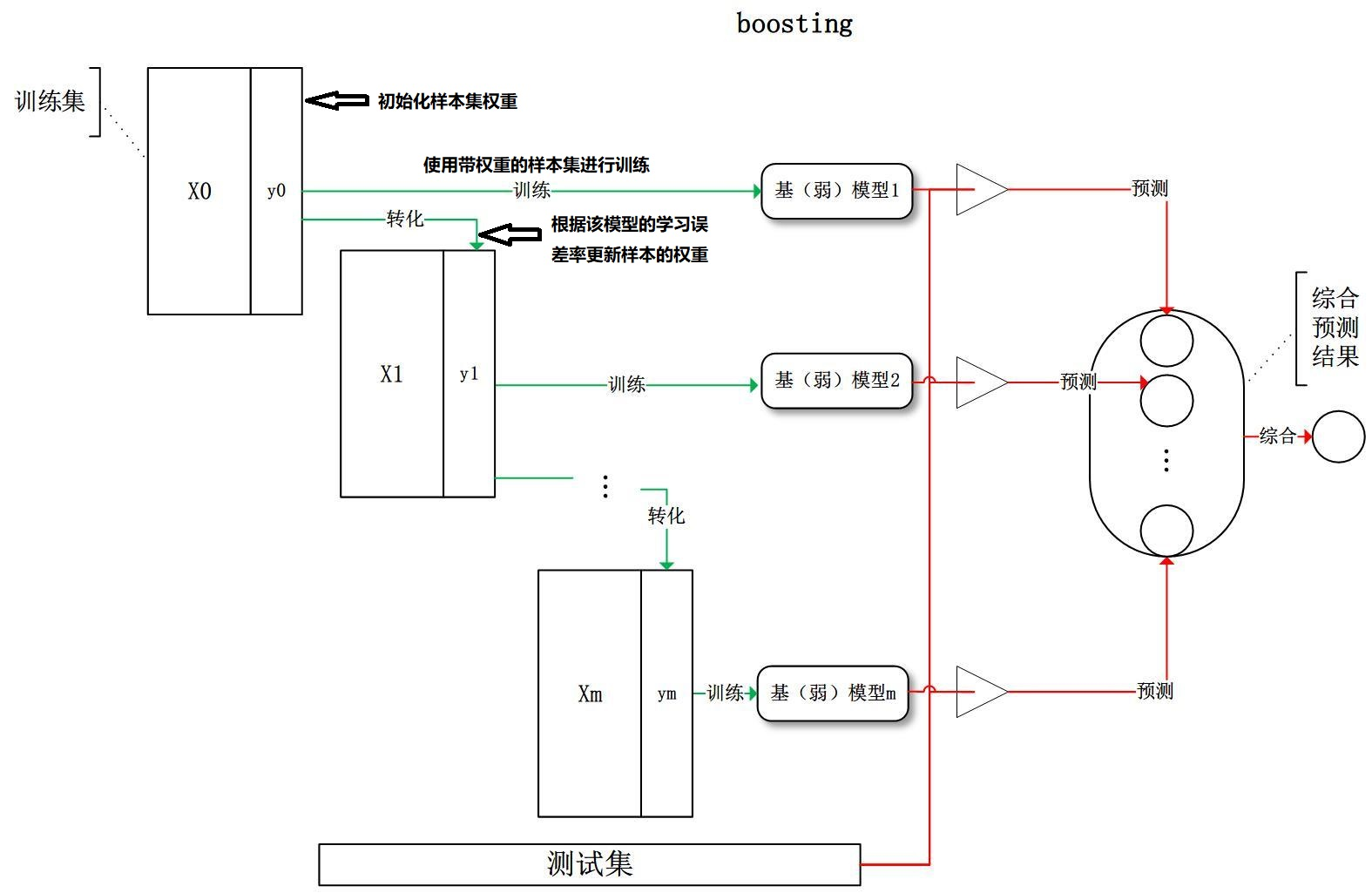Adaboost算法使用的是指数损失函数:$exp(-f(x)h(x))$

1. 初始化训练数据的权值分布。如果有N个样本，则每一个训练样本最开始时都被赋予相同的权值：1/N。
2. 训练弱分类器。具体训练过程中，如果某个样本点已经被准确地分类，那么在构造下一个训练集中，它的权值就被降低；相反，如果某个样本点没有被准确地分类，那么它的权值就得到提高。然后，权值更新过的样本集被用于训练下一个分类器，整个训练过程如此迭代地进行下去。 迭代的终止条件是强分类器的错误率达到某个预定的足够小的错误率或达到预先指定的最大迭代次数
3. 将各个训练得到的弱分类器组合成强分类器。各个弱分类器的训练过程结束后，加大分类误差率小的弱分类器的权重，使其在最终的分类函数中起着较大的决定作用，而降低分类误差率大的弱分类器的权重，使其在最终的分类函数中起着较小的决定作用。换言之，误差率低的弱分类器在最终分类器中占的权重较大，否则较小。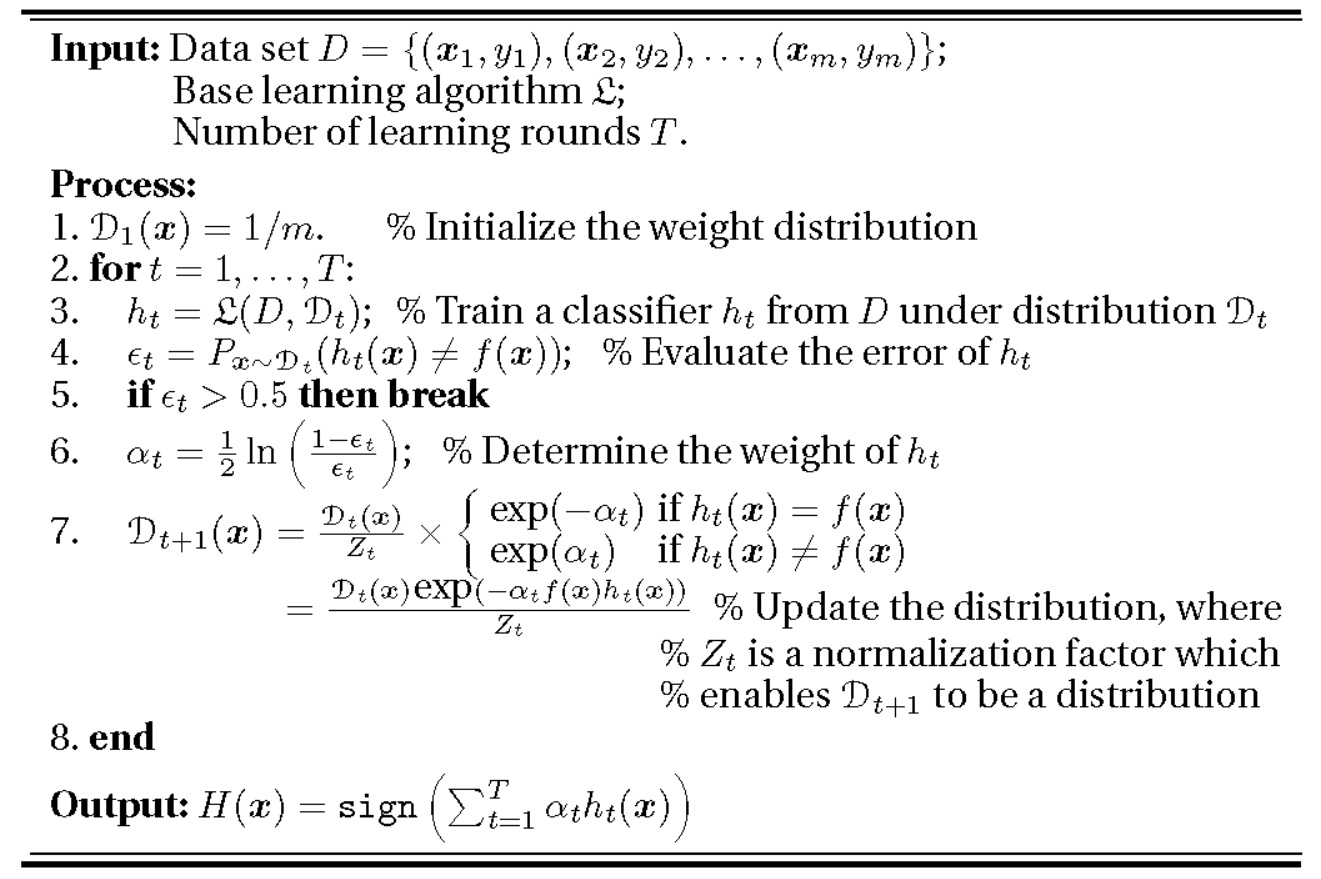$\epsilon_t=P(h_t(x) \neq f(x))=\sum_{h_t(x_i) \neq f(x_i)}w_{ti}$

$D_{t+1}(x)=(w_{t+1,1},...,w_{t+1,i},...,w_{t+1,N}) w_{t+1,i}=\begin{cases}\frac{w_{ti}}{Z_t}e^{-\alpha_t}&h_t(x)=f(x)\\\\ \frac{w_{ti}}{Z_t}e^{\alpha_t}&h_t(x)\neq f(x)\end{cases} Z_t=\sum\limits_{i=1}^Nw_{ti}exp(-\alpha_tf(x_i)h_{t}(x_i))$

### 2.2 提升树(Boosted Decision Tree)

$f_M(x)=\sum\limits_{m=1}^MT(x;\Theta_m)$

$f_m(x)=f_{m-1}(x)+T(x;\Theta_m)$

$\hat\Theta_m=\mathop{\arg\min}\limits_{\Theta_m}\sum\limits_{i=1}^NL(y_i,f_{m-1}(x_i)+T(x_i,\Theta_m))$

#### 2.2.2 二叉回归树

$T(x,\Theta)=\sum\limits_{j=1}^Jc_jI(x\in R_j)$

$f_0(x)=0 \\ f_m(x)=f_{m-1}(x)+T(x;\Theta_m),m=1,2,3,...,m \\ f_M(x)=\sum\limits_{m=1}^MT(x;\Theta_m)$

$\hat\Theta_m=\mathop{\arg\min}\limits_{\Theta_m}\sum\limits_{i=1}^NL(y_i,f_{m-1}(x_i)+T(x_i,\Theta_m))$

$L(y,f(x))=(y-f(x))^2$

$L(y_i,f_{m-1}(x)+T(x,\Theta_m))=[y-f_{m-1}(x)-T(x,\Theta_m)]^2=[r-T(x,\Theta_m)]^2$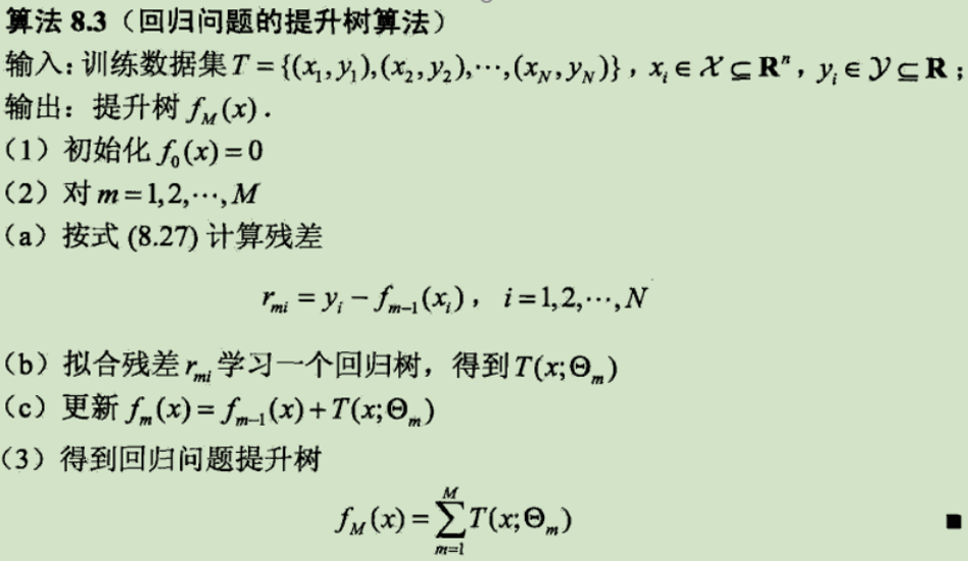### 2.3 梯度提升决策树(Gradient Boosting Decision Tree)

• Boosting: 这是一种迭代算法，每一次训练都是在前面已有模型的预测基础上进行。最简单地说，先训练一个初始模型，对比真实值和预测值的残差；用残差再训练一个模型，再计算残差；再训练。这样，每一个模型都专注于修正前面模型最不给力的效果方面。于是，通过这种方式联合多个弱分类器，就能得到一个强分类器。

$-\left[\dfrac{dL(y_i,f(x_i))}{df(x_i)}\right]_{f(x)=f_{m-1}(x)}\approx r_{mi}$## 3 Stacking

Stacking本质上是一种分层的结构，第一层通常是我们使用的不同基础模型，例如NN、决策树、KNN等。然后最后一层模型一般使用逻辑回归（LR）对这些模型进行进一步训练。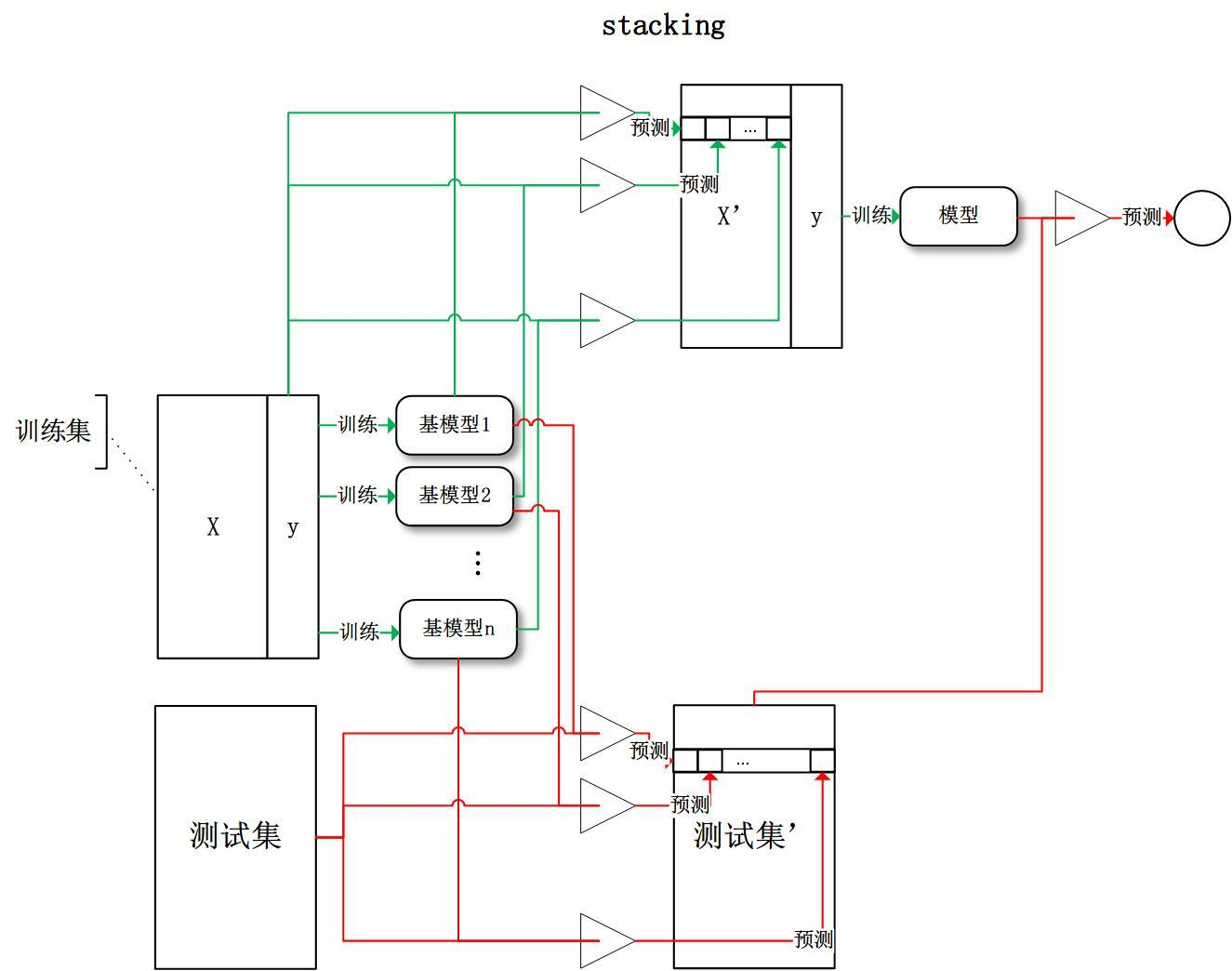## 4 总结

### 4.1 Bagging和Boosting的区别

1. 样本选择上：
• Bagging： 训练集是在原始集中有放回选取的，从原始集中选出的各轮训练集之间是独立的；
• Boosting： 每一轮的训练集不变，只是训练集中每个样例在分类器中的权重发生变化。而权值是根据上一轮的分类结果进行调整。
2. 样例权重：
• Bagging： 使用均匀取样，每个样例的权重相等
• Boosting： 根据错误率不断调整样例的权值，错误率越大则权重越大。
3. 预测函数：
• Bagging： 所有基分类器的权重相等
• Boosting： 每个弱分类器都有相应的权重，对于分类误差小的分类器会有更大的权重
4. 并行计算：
• Bagging： 各个预测函数可以并行生成；
• Boosting： 理论上各个预测函数只能顺序生成，因为后一个模型参数需要前一轮模型的结果。计算角度来看，两种方法都可以并行。
5. Bagging是减少variance(方差)，而Boosting是减少bias(偏差)

### 4.2 Random Forest与Bagging的区别

1. 输入样本数目： Random Forest是选与输入样本的数目相同多的次数（可能一个样本会被选取多次，同时也会造成一些样本不会被选取到），而Bagging一般选取比输入样本的数目少的样本；
2. 特征数目： Bagging是用全部特征来得到分类器，而Random Forest是需要从全部特征中选取其中的一部分来训练得到分类器； 一般Random Forest效果比Bagging效果好！

### 4.3 GBDT和随机森林的对比

1. 都是由多棵树组成
2. 最终的结果都是由多棵树一起决定

1. 组成随机森林的树可以是分类树，也可以是回归树；而GBDT只由回归树组成
2. 组成随机森林的树可以并行生成；而GBDT只能是串行生成
3. 对于最终的输出结果而言，随机森林采用多数投票等；而GBDT则是将所有结果累加起来，或者加权累加起来
4. 随机森林对异常值不敏感，GBDT对异常值非常敏感
5. 随机森林对训练集一视同仁，GBDT是基于权值的弱分类器的集成
6. 随机森林是通过减少模型方差提高性能，GBDT是通过减少模型偏差提高性能